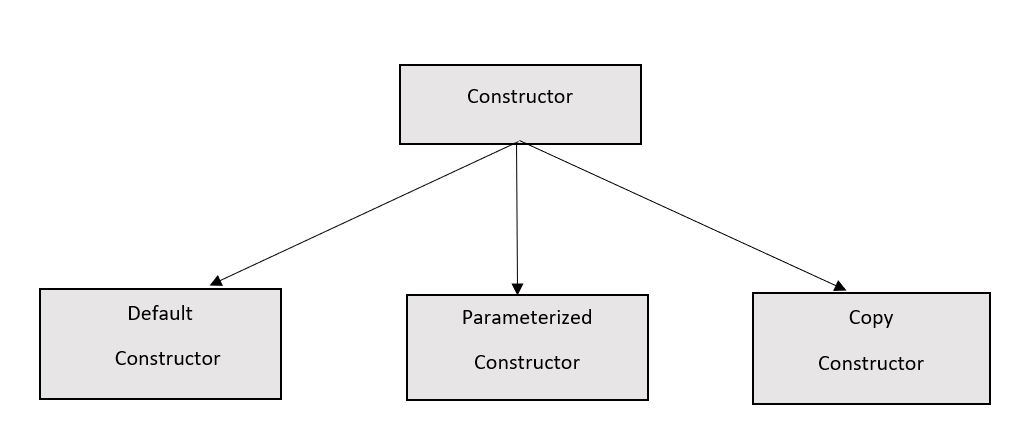×

Search anything:

# constructor in C++

#### C++ Software Engineering constructor oopA constructor is a member function of a class which initializes objects of a class. In C++, Constructor is automatically called when object(instance of class) is created it is considered to be a special member function of the class.

Syntax :

``````classname( function parameters )
{
function body
}
``````

### How constructors are different from a normal member function?

A constructor is different from normal functions in following ways:

1. Constructor has same name as the class itself
2. Constructors don’t have return type
3. A constructor is automatically called when an object is created.
4. If we do not specify a constructor, C++ compiler generates a default constructor for us (expects no parameters and has an empty body).

### Types of Constructors### 1) Default Constructors

Default constructor is the constructor which doesn’t take any argument. It has no parameters.

``````// Cpp program to illustrate the
// concept of Constructors
#include <iostream>
using namespace std;

class construct {
public:
int a, b;

// Default Constructor
construct()
{
a = 10;
b = 20;
}
};

int main()
{
// Default constructor called automatically
// when the object is created
construct c;
cout << "a: " << c.a << endl
<< "b: " << c.b;
return 1;
}
``````

Output:

``````a: 10
b: 20
``````

Note: Even if we do not define any constructor explicitly, the compiler will automatically provide a default constructor implicitly.

### 2) Parameterized Constructors

It is possible to pass arguments to constructors. Typically, these arguments help initialize an object when it is created. To create a parameterized constructor, simply add parameters to it the way you would to any other function. When you define the constructor’s body, use the parameters to initialize the object.

``````// CPP program to illustrate
// parameterized constructors
#include <iostream>
using namespace std;

class Point {
private:
int x, y;

public:
// Parameterized Constructor
Point(int x1, int y1)
{
x = x1;
y = y1;
}

int getX()
{
return x;
}
int getY()
{
return y;
}
};

int main()
{
// Constructor called
Point p1(10, 15);

// Access values assigned by constructor
cout << "p1.x = " << p1.getX() << ", p1.y = " << p1.getY();

return 0;
}
``````

Output:

``````p1.x = 10, p1.y = 15
``````

When an object is declared in a parameterized constructor, the initial values have to be passed as arguments to the constructor function. The normal way of object declaration may not work. The constructors can be called explicitly or implicitly.

`````` Example e = Example(0, 50); // Explicit call

Example e(0, 50);           // Implicit call
``````

### Uses of Parameterized constructor

1. It is used to initialize the various data elements of different objects with different values when they are created.
2. It is used to overload constructors.

### 3) Copy Constructors

A copy constructor is a member function which initializes an object using another object of the same class. A copy constructor has the following general function prototype:

``````    ClassName (const ClassName &old_obj);
``````

Code example:

``````#include<iostream>
using namespace std;

class Point
{
private:
int x, y;
public:
Point(int x1, int y1) { x = x1; y = y1; }

// Copy constructor
Point(const Point &p2) {x = p2.x; y = p2.y; }

int getX()            {  return x; }
int getY()            {  return y; }
};

int main()
{
Point p1(10, 15); // Normal constructor is called here
Point p2 = p1; // Copy constructor is called here

// Let us access values assigned by constructors
cout << "p1.x = " << p1.getX() << ", p1.y = " << p1.getY();
cout << "\np2.x = " << p2.getX() << ", p2.y = " << p2.getY();

return 0;
}
``````

Output:

``````p1.x = 10, p1.y = 15
p2.x = 10, p2.y = 15
``````

### When is copy constructor called ?

In C++, a Copy Constructor may be called in following cases:

1. When an object of the class is returned by value.
2. When an object of the class is passed (to a function) by value as an argument.
3. When an object is constructed based on another object of the same class.
4. When the compiler generates a temporary object.

It is, however, not guaranteed that a copy constructor will be called in all these cases, because the C++ Standard allows the compiler to optimize the copy away in certain cases, one example is the return value optimization.

### Question

Consider the following C++ code:

``````#include<iostream>
using namespace std;
class Point {
Point() { cout << "Constructor called"; }
};

int main()
{
Point t1;
return 0;
}
``````

#### What is the output of the above program?

Compile Error
Run Time error
Constructor Call
Depends on operating system
By default all members of a class are private. Since no access specifier is there for Point(), it becomes private and it is called outside the class when t1 is constructed in main.#### Harshita Sahai

Maintainer at OpenGenus | Previously Software Developer, Intern at OpenGenus (June to August 2019) | B.Tech in Information Technology from Guru Gobind Singh Indraprastha University (2017 to 2021)